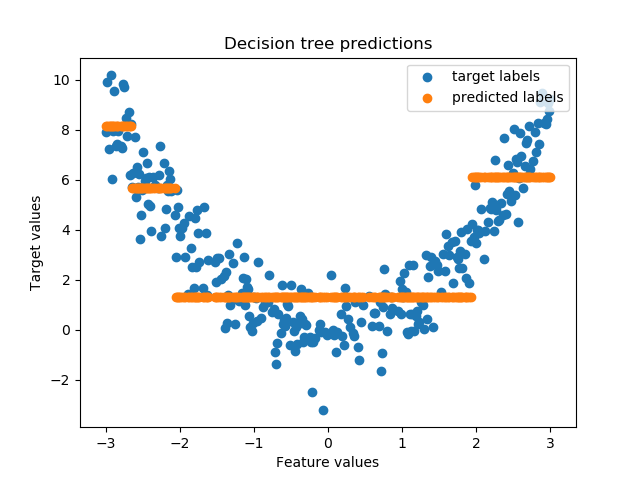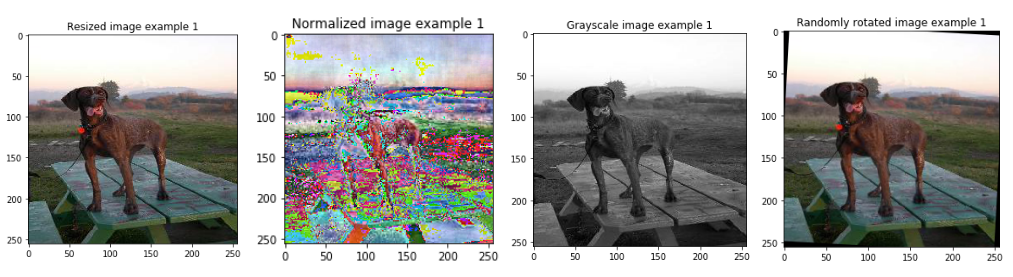# Machine learning basics

This repository contains implementations of basic machine learning algorithms in plain Python (Python Version 3.6+). All algorithms are implemented from scratch without using additional machine learning libraries. The intention of these notebooks is to provide a basic understanding of the algorithms and their underlying structure, not to provide the most efficient implementations.## Data preprocessing

After several requests I started preparing notebooks on how to preprocess datasets for machine learning. Within the next months I will add one notebook for each kind of dataset (text, images, ...). As before, the intention of these notebooks is to provide a basic understanding of the preprocessing steps, not to provide the most efficient implementations.## Live demo

Run the notebooks online without having to clone the repository or install jupyter:.

Note: this does not work for the `data_preprocessing.ipynb` and `image_preprocessing.ipynb` notebooks because they require downloading a dataset first.

## Feedback

If you have a favorite algorithm that should be included or spot a mistake in one of the notebooks, please let me know by creating a new issue.

Get A Weekly Email With Trending Projects For These Topics
No Spam. Unsubscribe easily at any time.
Python (888,878
Jupyter Notebook (178,660
Learning (75,671
Machine Learning (40,833
Algorithms (38,014
Neural Network (16,097
Basic (10,672
Preprocessing (2,298
Machine Learning Algorithms (2,178
Linear Regression (1,779
Logistic Regression (1,655
Kmeans (1,108
Perceptron (984
Ipynb (599
Knn (443
K Nearest Neighbours (91
K Nearest Neighbors (67
Python Implementations (11